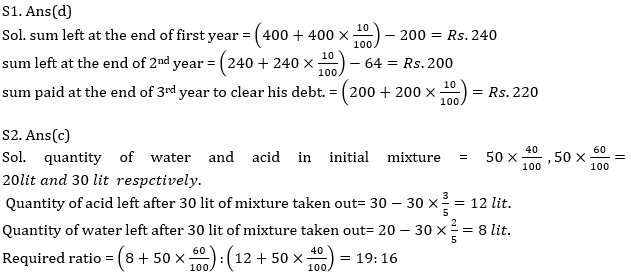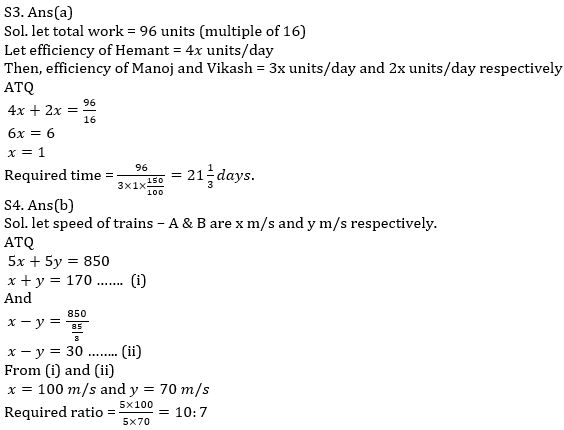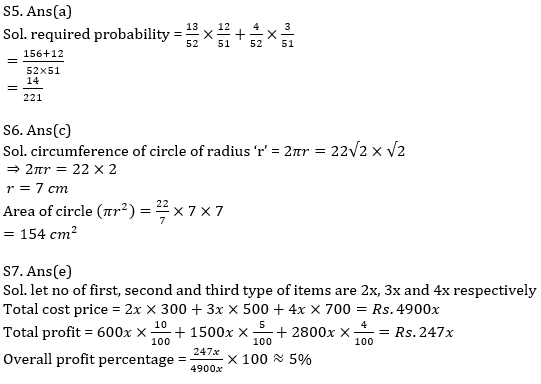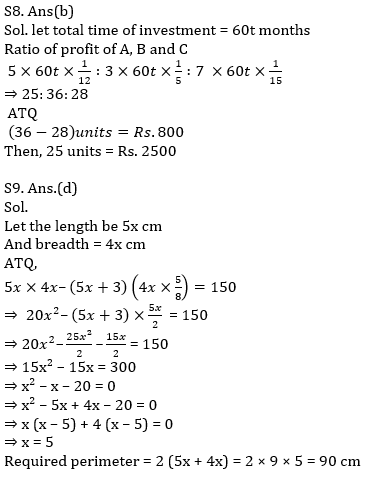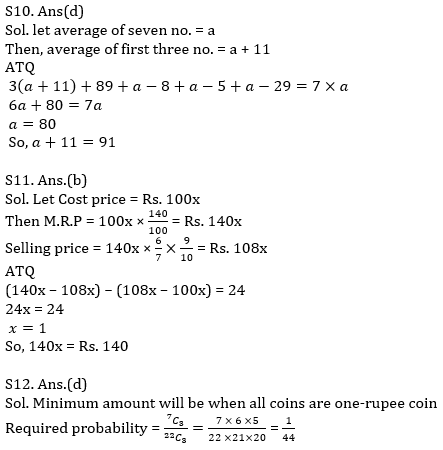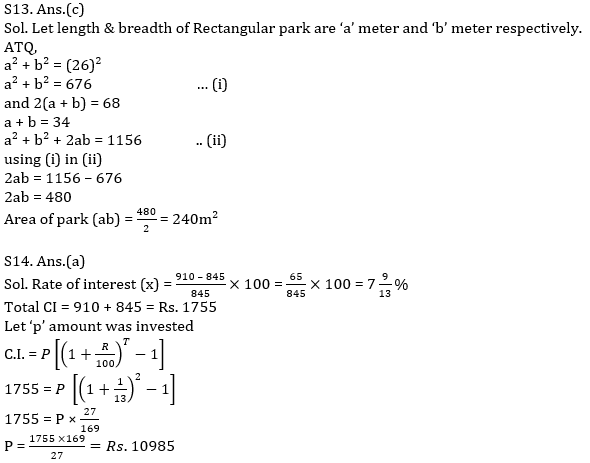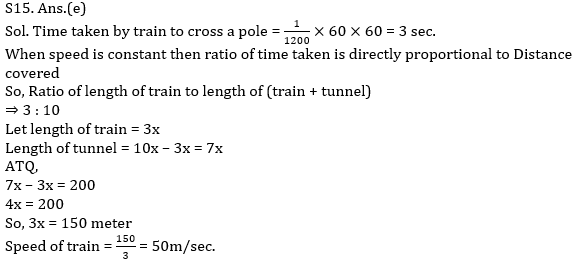Latest Banking jobs   »   Quantitative Aptitude Quiz For IBPS RRB...

# Quantitative Aptitude Quiz For IBPS RRB PO, Clerk Prelims 2021- 2nd August

Q1. Harsh borrow Rs. 400 at 10% rate of interest. He paid Rs.200 and Rs.64 at the end of 1st and 2nd year respectively. Then find how much money he will pay at the end of 3rd year to clear his debt?
(a) Rs. 200
(b) Rs. 240
(c) Rs. 264
(d) Rs. 220
(e) Rs. can’t be determined.

Q2. A container has mixture of water and acid in which water is 40% out of total mixture of 50 lit. if 30 lit of the mixture is taken out and 50 lit of another mixture of water and acid is added and in second mixture acid is 40%, then find ratio of water to acid in final mixture.
(a) 16:19
(b) 8:7
(c) 19:16
(d) 7:8
(e) 17:19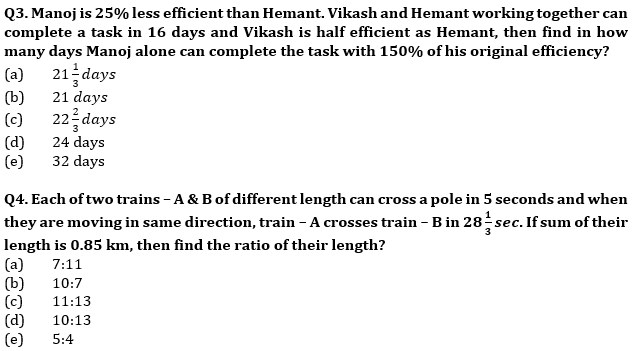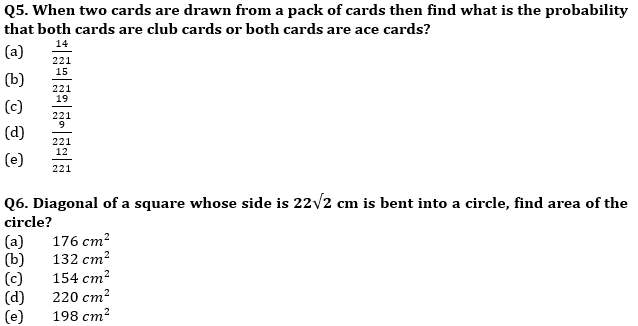Q7. A man purchases three type of item in ratio 2:3:4 and their cost price are Rs. 300, Rs. 500 and Rs. 700 respectively. If he sold first, second and third type of article at 10%, 5% and 4% profit respectively, then find his overall approximate profit percentage?
(a) 7%
(b) 2%
(c) 4%
(d) 9%
(e) 5%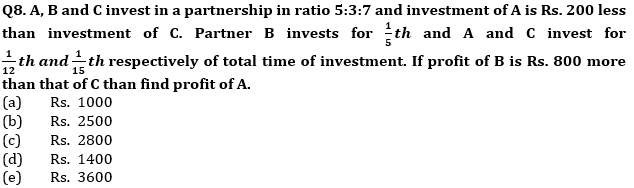Q9. The sides of a rectangle are in the ratio of 5 : 4 (length : breadth). If the length is increased by 3 cm and breadth is reduced by 37½%, the new area obtained is 150 cm² less than the original area. Find the perimeter of the rectangle?
(a) 72 cm
(b) 108 cm
(c) 126 cm
(d) 90 cm
(e) 100 cm

Q10. Average of first three no. out of seven no. is 11 more than average of seven no. and 5th , 6th and 7th no. are 8, 5 and 29 less than average of seven number respectively. If 4th no. is 89, then find average of initial three no.
(a) 80
(b) 70
(c) 81
(d) 91
(e) 61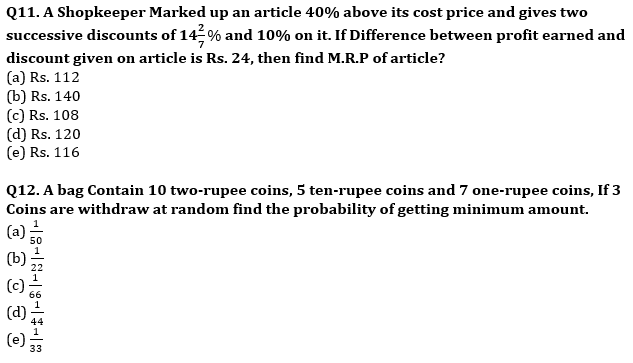Q13. Diagonal of rectangular park is 26 meter and perimeter of rectangular park is 68 meter Find area of the park?
(a) 120m²
(b) 180m²
(c) 240m²
(d) 60m²
(e) None of these

Q14. Manoj invested a sum at x% per annum at C.I. If first year and second year C.I on that sum is Rs. 845 and Rs. 910 Find Amount invested?
(a) Rs. 10985
(b) Rs. 10000
(c) Rs. 13000
(d) Rs. 10900
(e) Rs. 13985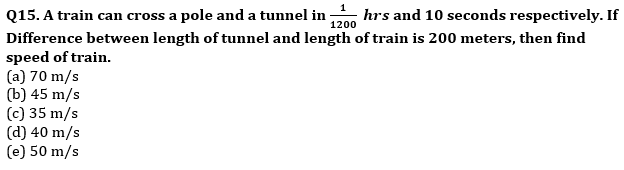Practice More Questions of Quantitative Aptitude for Competitive Exams:

###### Target IBPS RRB PO and Clerk Prelims 2021

Solutions